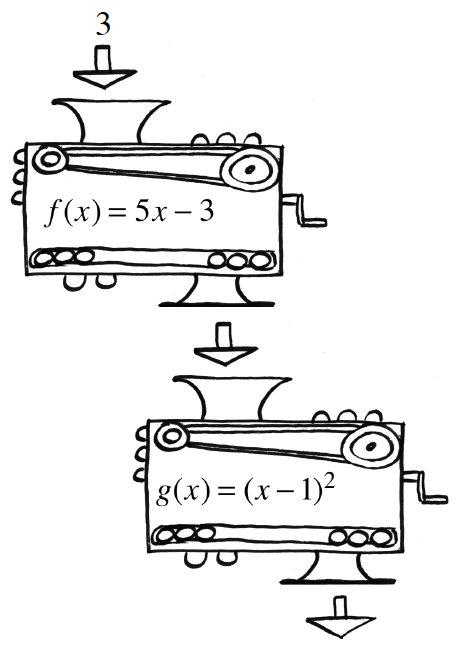### Home > CCA2 > Chapter 5 > Lesson 5.1.3 > Problem5-48

5-48.Two function machines, $f(x)=5x−3$ and $g(x)=(x−1)^2$, are shown at right.

1. Suppose $f(3)$, (not $x=3$), is dropped into the $g(x)$ machine. This is written as $g(f(3))$. What is this output?

$f(3)=12$
$\text{so}\ g(f(3))=g(12)=121$

2. Using the same function machines, what is $f(g(3))$? Be careful! The result is different from the last one because the order in which you use the machines has been switched! With $f(g(3))$, first you find $g(3)$, then you substitute that answer into the machine named $f(x)$ .

$f(g(3))=17$